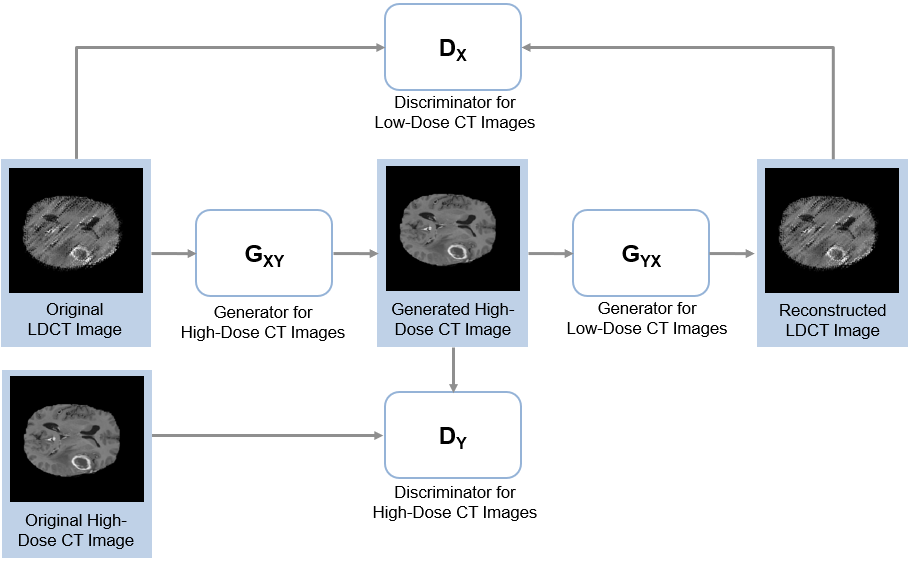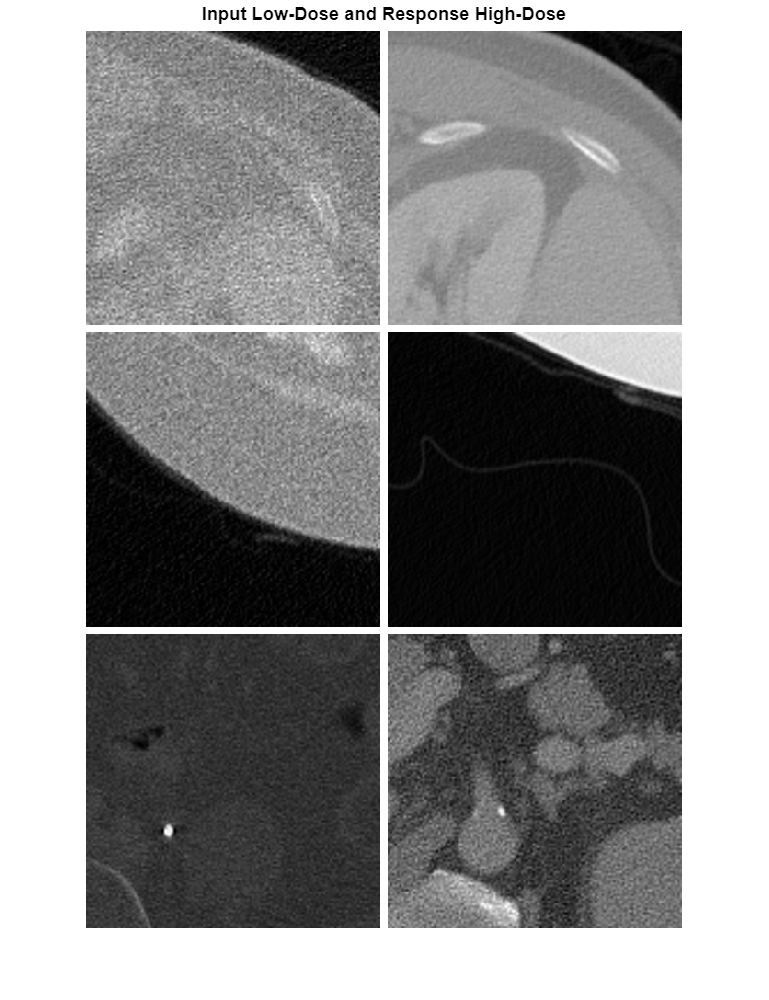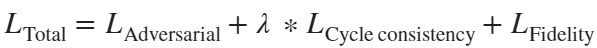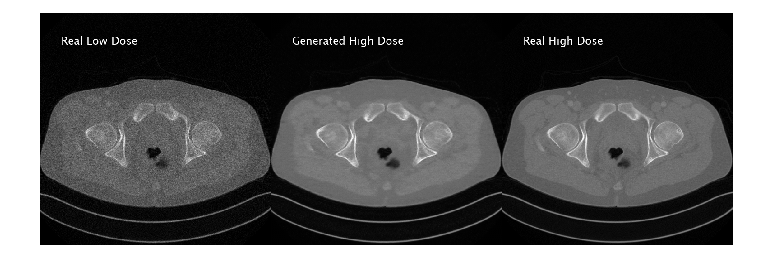# Unsupervised Medical Image Denoising Using CycleGAN

This example shows how to generate high-quality high-dose computed tomography (CT) images from noisy low-dose CT images using a CycleGAN neural network.

Note: This example references the Low Dose CT Grand Challenge data set, as accessed on May 1, 2021. The example uses chest images from the data set that are now under restricted access. To run this example, you must have a compatible data set with low-dose and high-dose CT images, and adapt the data preprocessing and training options to suit your data.

X-ray CT is a popular imaging modality used in clinical and industrial applications because it produces high-quality images and offers superior diagnostic capabilities. To protect the safety of patients, clinicians recommend a low radiation dose. However, a low radiation dose results in a lower signal-to-noise ratio (SNR) in the images, and therefore reduces the diagnostic accuracy.

Deep learning techniques can improve the image quality for low-dose CT (LDCT) images. Using a generative adversarial network (GAN) for image-to-image translation, you can convert noisy LDCT images to images of the same quality as regular-dose CT images. For this application, the source domain consists of LDCT images and the target domain consists of regular-dose images. For more information, see Get Started with GANs for Image-to-Image Translation (Image Processing Toolbox).

CT image denoising requires a GAN that performs unsupervised training because clinicians do not typically acquire matching pairs of low-dose and regular-dose CT images of the same patient in the same session. This example uses a cycle-consistent GAN (CycleGAN) trained on patches of image data from a large sample of data. For a similar approach using a UNIT neural network trained on full images from a limited sample of data, see Unsupervised Medical Image Denoising Using UNIT (Image Processing Toolbox).This example uses data from the Low Dose CT Grand Challenge [2, 3, 4]. The data includes pairs of regular-dose CT images and simulated low-dose CT images for 99 head scans (labeled N for neuro), 100 chest scans (labeled C for chest), and 100 abdomen scans (labeled L for liver). The size of the data set is 1.2 TB.

Specify `dataDir` as the desired location of the data set.

`dataDir = fullfile(tempdir,"LDCT","LDCT-and-Projection-data");`

To download the data, go to the Cancer Imaging Archive website. This example uses only images from the chest. Download the chest files from the "Images (DICOM, 952 GB)" data set into the directory specified by `dataDir` using the NBIA Data Retriever. When the download is successful, `dataDir` contains 50 subfolders with names such as "C002" and "C004", ending with "C296".

### Create Datastores for Training, Validation, and Testing

The LDCT data set provides pairs of low-dose and high-dose CT images. However, the CycleGAN architecture requires unpaired data for unsupervised learning. This example simulates unpaired training and validation data by partitioning images such that the patients used to obtain low-dose CT and high-dose CT images do not overlap. The example retains pairs of low-dose and regular-dose images for testing.

Split the data into training, validation, and test data sets using the `createLDCTFolderList` helper function. This function is attached to the example as a supporting file. The helper function splits the data such that there is roughly good representation of the two types of images in each group. Approximately 80% of the data is used for training, 15% is used for testing, and 5% is used for validation.

```maxDirsForABodyPart = 25; [filesTrainLD,filesTrainHD,filesTestLD,filesTestHD,filesValLD,filesValHD] = ... createLDCTFolderList(dataDir,maxDirsForABodyPart);```

Create image datastores that contain training and validation images for both domains, namely low-dose CT images and high-dose CT images. The data set consists of DICOM images, so use the custom `ReadFcn` name-value argument in `imageDatastore` to enable reading the data.

```exts = ".dcm"; readFcn = @(x)dicomread(x); imdsTrainLD = imageDatastore(filesTrainLD,FileExtensions=exts,ReadFcn=readFcn); imdsTrainHD = imageDatastore(filesTrainHD,FileExtensions=exts,ReadFcn=readFcn); imdsValLD = imageDatastore(filesValLD,FileExtensions=exts,ReadFcn=readFcn); imdsValHD = imageDatastore(filesValHD,FileExtensions=exts,ReadFcn=readFcn); imdsTestLD = imageDatastore(filesTestLD,FileExtensions=exts,ReadFcn=readFcn); imdsTestHD = imageDatastore(filesTestHD,FileExtensions=exts,ReadFcn=readFcn);```

The number of low-dose and high-dose images can differ. Select a subset of the files such that the number of images is equal.

```numTrain = min(numel(imdsTrainLD.Files),numel(imdsTrainHD.Files)); imdsTrainLD = subset(imdsTrainLD,1:numTrain); imdsTrainHD = subset(imdsTrainHD,1:numTrain); numVal = min(numel(imdsValLD.Files),numel(imdsValHD.Files)); imdsValLD = subset(imdsValLD,1:numVal); imdsValHD = subset(imdsValHD,1:numVal); numTest = min(numel(imdsTestLD.Files),numel(imdsTestHD.Files)); imdsTestLD = subset(imdsTestLD,1:numTest); imdsTestHD = subset(imdsTestHD,1:numTest);```

### Preprocess and Augment Data

Preprocess the data by using the `transform` function with custom preprocessing operations specified by the `normalizeCTImages` helper function. This function is attached to the example as a supporting file. The `normalizeCTImages` function rescales the data to the range [-1, 1].

```timdsTrainLD = transform(imdsTrainLD,@(x){normalizeCTImages(x)}); timdsTrainHD = transform(imdsTrainHD,@(x){normalizeCTImages(x)}); timdsValLD = transform(imdsValLD,@(x){normalizeCTImages(x)}); timdsValHD = transform(imdsValHD,@(x){normalizeCTImages(x)}); timdsTestLD = transform(imdsTestLD,@(x){normalizeCTImages(x)}); timdsTestHD = transform(imdsTestHD,@(x){normalizeCTImages(x)});```

Combine the low-dose and high-dose training data by using a `randomPatchExtractionDatastore` (Image Processing Toolbox). When reading from this datastore, augment the data using random rotation and horizontal reflection.

```inputSize = [128,128,1]; augmenter = imageDataAugmenter(RandRotation=@()90*(randi([0,1],1)),RandXReflection=true); dsTrain = randomPatchExtractionDatastore(timdsTrainLD,timdsTrainHD, ... inputSize(1:2),PatchesPerImage=16,DataAugmentation=augmenter);```

Combine the validation data by using a `randomPatchExtractionDatastore`. You do not need to perform augmentation when reading validation data.

`dsVal = randomPatchExtractionDatastore(timdsValLD,timdsValHD,inputSize(1:2));`

#### Visualize Data Set

Look at a few low-dose and high-dose image patch pairs from the training set. Notice that the image pairs of low-dose (left) and high-dose (right) images are unpaired, as they are from different patients.

```numImagePairs = 6; imagePairsTrain = []; for i = 1:numImagePairs imLowAndHighDose = read(dsTrain); inputImage = imLowAndHighDose.InputImage{1}; inputImage = rescale(im2single(inputImage)); responseImage = imLowAndHighDose.ResponseImage{1}; responseImage = rescale(im2single(responseImage)); imagePairsTrain = cat(4,imagePairsTrain,inputImage,responseImage); end montage(imagePairsTrain,Size=[numImagePairs 2],BorderSize=4,BackgroundColor="w")```#### Batch Training and Validation Data During Training

This example uses a custom training loop. The `minibatchqueue` object is useful for managing the mini-batching of observations in custom training loops. The `minibatchqueue` object also casts data to a `dlarray` object that enables auto differentiation in deep learning applications.

Process the mini-batches by concatenating image patches along the batch dimension using the helper function `concatenateMiniBatchLD2HDCT`. This function is attached to the example as a supporting file. Specify the mini-batch data extraction format as "`SSCB"` (spatial, spatial, channel, batch). Discard any partial mini-batches with less than `miniBatchSize` observations.

```miniBatchSize = 32; mbqTrain = minibatchqueue(dsTrain, ... MiniBatchSize=miniBatchSize, ... MiniBatchFcn=@concatenateMiniBatchLD2HDCT, ... PartialMiniBatch="discard", ... MiniBatchFormat="SSCB"); mbqVal = minibatchqueue(dsVal, ... MiniBatchSize=miniBatchSize, ... MiniBatchFcn=@concatenateMiniBatchLD2HDCT, ... PartialMiniBatch="discard", ... MiniBatchFormat="SSCB");```

### Create Generator and Discriminator Networks

The CycleGAN consists of two generators and two discriminators. The generators perform image-to-image translation from low-dose to high-dose and vice versa. The discriminators are PatchGAN networks that return the patch-wise probability that the input data is real or generated. One discriminator distinguishes between the real and generated low-dose images and the other discriminator distinguishes between real and generated high-dose images.

Create each generator network using the `cycleGANGenerator` (Image Processing Toolbox) function. For an input size of 256-by-256 pixels, specify the `NumResidualBlocks` argument as `9`. By default, the function has 3 encoder modules and uses 64 filters in the first convolutional layer.

```numResiduals = 6; genHD2LD = cycleGANGenerator(inputSize,NumResidualBlocks=numResiduals,NumOutputChannels=1); genLD2HD = cycleGANGenerator(inputSize,NumResidualBlocks=numResiduals,NumOutputChannels=1);```

Create each discriminator network using the `patchGANDiscriminator` (Image Processing Toolbox) function. Use the default settings for the number of downsampling blocks and number of filters in the first convolutional layer in the discriminators.

```discLD = patchGANDiscriminator(inputSize); discHD = patchGANDiscriminator(inputSize);```

### Define Loss Functions and Scores

The `modelGradients` helper function calculates the gradients and losses for the discriminators and generators. This function is defined in the Supporting Functions section of this example.

The objective of the generator is to generate translated images that the discriminators classify as real. The generator loss is a weighted sum of three types of losses: adversarial loss, cycle consistency loss, and fidelity loss. Fidelity loss is based on structural similarity (SSIM) loss.

`${\mathit{L}}_{\mathrm{Total}}^{}={\mathit{L}}_{\mathrm{Adversarial}}^{}+\lambda \text{\hspace{0.17em}}*{\mathit{L}}_{\mathrm{Cycle}\text{\hspace{0.17em}}\mathrm{consistency}}^{}+{\mathit{L}}_{\mathrm{Fidelity}}^{}$`

Specify the weighting factor $\lambda$ that controls the relative significance of the cycle consistency loss with the adversarial and fidelity losses.

`lambda = 10;`

The objective of each discriminator is to correctly distinguish between real images (1) and translated images (0) for images in its domain. Each discriminator has a single loss function that relies on the mean squared error (MSE) between the expected and predicted output.

### Specify Training Options

Train with a mini-batch size of 32 for 3 epochs.

```numEpochs = 3; miniBatchSize = 32;```

Specify the options for Adam optimization. For both generator and discriminator networks, use:

• A learning rate of 0.0002

• A gradient decay factor of 0.5

• A squared gradient decay factor of 0.999

```learnRate = 0.0002; gradientDecay = 0.5; squaredGradientDecayFactor = 0.999;```

Initialize Adam parameters for the generators and discriminators.

```avgGradGenLD2HD = []; avgSqGradGenLD2HD = []; avgGradGenHD2LD = []; avgSqGradGenHD2LD = []; avgGradDiscLD = []; avgSqGradDiscLD = []; avgGradDiscHD = []; avgSqGradDiscHD = [];```

Display the generated validation images every 100 iterations.

`validationFrequency = 100;`

By default, the example downloads a pretrained version of the CycleGAN generator for low-dose to high-dose CT. The pretrained network enables you to run the entire example without waiting for training to complete.

To train the network, set the `doTraining` variable in the following code to `true`. Train the model in a custom training loop. For each iteration:

• Read the data for the current mini-batch using the `next` function.

• Evaluate the model gradients using the `dlfeval` function and the `modelGradients` helper function.

• Update the network parameters using the `adamupdate` function.

• Display the input and translated images for both the source and target domains after each epoch.

Train on a GPU if one is available. Using a GPU requires Parallel Computing Toolbox™ and a CUDA® enabled NVIDIA® GPU. For more information, see GPU Computing Requirements (Parallel Computing Toolbox). Training takes about 30 hours on an NVIDIA™ Titan X with 24 GB of GPU memory.

```doTraining = false; if doTraining iteration = 0; start = tic; % Create a directory to store checkpoints checkpointDir = fullfile(dataDir,"checkpoints"); if ~exist(checkpointDir,"dir") mkdir(checkpointDir); end % Initialize plots for training progress [figureHandle,tileHandle,imageAxes,scoreAxesX,scoreAxesY, ... lineScoreGenLD2HD,lineScoreGenD2LD,lineScoreDiscHD,lineScoreDiscLD] = ... initializeTrainingPlotLD2HDCT_CycleGAN; for epoch = 1:numEpochs shuffle(mbqTrain); % Loop over mini-batches while hasdata(mbqTrain) iteration = iteration + 1; % Read mini-batch of data [imageLD,imageHD] = next(mbqTrain); % Convert mini-batch of data to dlarray and specify the dimension labels % "SSCB" (spatial, spatial, channel, batch) imageLD = dlarray(imageLD,"SSCB"); imageHD = dlarray(imageHD,"SSCB"); % If training on a GPU, then convert data to gpuArray if canUseGPU imageLD = gpuArray(imageLD); imageHD = gpuArray(imageHD); end % Calculate the loss and gradients [genHD2LDGrad,genLD2HDGrad,discrXGrad,discYGrad, ... genHD2LDState,genLD2HDState,scores,imagesOutLD2HD,imagesOutHD2LD] = ... dlfeval(@modelGradients,genLD2HD,genHD2LD, ... discLD,discHD,imageHD,imageLD,lambda); genHD2LD.State = genHD2LDState; genLD2HD.State = genLD2HDState; % Update parameters of discLD, which distinguishes % the generated low-dose CT images from real low-dose CT images [discLD.Learnables,avgGradDiscLD,avgSqGradDiscLD] = ... adamupdate(discLD.Learnables,discrXGrad,avgGradDiscLD, ... avgSqGradDiscLD,iteration,learnRate,gradientDecay,squaredGradientDecayFactor); % Update parameters of discHD, which distinguishes % the generated high-dose CT images from real high-dose CT images [discHD.Learnables,avgGradDiscHD,avgSqGradDiscHD] = ... adamupdate(discHD.Learnables,discYGrad,avgGradDiscHD, ... avgSqGradDiscHD,iteration,learnRate,gradientDecay,squaredGradientDecayFactor); % Update parameters of genHD2LD, which % generates low-dose CT images from high-dose CT images [genHD2LD.Learnables,avgGradGenHD2LD,avgSqGradGenHD2LD] = ... adamupdate(genHD2LD.Learnables,genHD2LDGrad,avgGradGenHD2LD, ... avgSqGradGenHD2LD,iteration,learnRate,gradientDecay,squaredGradientDecayFactor); % Update parameters of genLD2HD, which % generates high-dose CT images from low-dose CT images [genLD2HD.Learnables,avgGradGenLD2HD,avgSqGradGenLD2HD] = ... adamupdate(genLD2HD.Learnables,genLD2HDGrad,avgGradGenLD2HD, ... avgSqGradGenLD2HD,iteration,learnRate,gradientDecay,squaredGradientDecayFactor); % Update the plots of network scores updateTrainingPlotLD2HDCT_CycleGAN(scores,iteration,epoch,start,scoreAxesX,scoreAxesY,... lineScoreGenLD2HD,lineScoreGenD2LD, ... lineScoreDiscHD,lineScoreDiscLD) % Every validationFrequency iterations, display a batch of % generated images using the held-out generator input if mod(iteration,validationFrequency) == 0 || iteration == 1 displayGeneratedLD2HDCTImages(mbqVal,imageAxes,genLD2HD,genHD2LD); end end % Save the model after each epoch if canUseGPU [genLD2HD,genHD2LD,discLD,discHD] = ... gather(genLD2HD,genHD2LD,discLD,discHD); end generatorHighDoseToLowDose = genHD2LD; generatorLowDoseToHighDose = genLD2HD; discriminatorLowDose = discLD; discriminatorHighDose = discHD; modelDateTime = string(datetime("now",Format="yyyy-MM-dd-HH-mm-ss")); save(checkpointDir+filesep+"LD2HDCTCycleGAN-"+modelDateTime+"-Epoch-"+epoch+".mat", ... 'generatorLowDoseToHighDose','generatorHighDoseToLowDose', ... 'discriminatorLowDose','discriminatorHighDose'); end % Save the final model modelDateTime = string(datetime("now",Format="yyyy-MM-dd-HH-mm-ss")); save(checkpointDir+filesep+"trainedLD2HDCTCycleGANNet-"+modelDateTime+".mat", ... 'generatorLowDoseToHighDose','generatorHighDoseToLowDose', ... 'discriminatorLowDose','discriminatorHighDose'); else net_url = "https://www.mathworks.com/supportfiles/vision/data/trainedLD2HDCTCycleGANNet.mat"; downloadTrainedNetwork(net_url,dataDir); load(fullfile(dataDir,"trainedLD2HDCTCycleGANNet.mat")); end```

### Generate New Images Using Test Data

Define the number of test images to use for calculating quality metrics. Randomly select two test images to display.

```numTest = timdsTestLD.numpartitions; numImagesToDisplay = 2; idxImagesToDisplay = randi(numTest,1,numImagesToDisplay);```

Initialize variables to calculate PSNR and SSIM.

```origPSNR = zeros(numTest,1); generatedPSNR = zeros(numTest,1); origSSIM = zeros(numTest,1); generatedSSIM = zeros(numTest,1);```

To generate new translated images, use the `predict` function. Read images from the test data set and use the trained generators to generate new images.

```for idx = 1:numTest imageTestLD = read(timdsTestLD); imageTestHD = read(timdsTestHD); imageTestLD = cat(4,imageTestLD{1}); imageTestHD = cat(4,imageTestHD{1}); % Convert mini-batch of data to dlarray and specify the dimension labels % "SSCB" (spatial, spatial, channel, batch) imageTestLD = dlarray(imageTestLD,"SSCB"); imageTestHD = dlarray(imageTestHD,"SSCB"); % If running on a GPU, then convert data to gpuArray if canUseGPU imageTestLD = gpuArray(imageTestLD); imageTestHD = gpuArray(imageTestHD); end % Generate translated images generatedImageHD = predict(generatorLowDoseToHighDose,imageTestLD); generatedImageLD = predict(generatorHighDoseToLowDose,imageTestHD); % Display a few images to visualize the network responses if ismember(idx,idxImagesToDisplay) figure origImLD = rescale(extractdata(imageTestLD)); genImHD = rescale(extractdata(generatedImageHD)); montage({origImLD,genImHD},Size=[1 2],BorderSize=5) title("Original LDCT Test Image "+idx+" (Left), Generated HDCT Image (Right)") end origPSNR(idx) = psnr(imageTestLD,imageTestHD); generatedPSNR(idx) = psnr(generatedImageHD,imageTestHD); origSSIM(idx) = multissim(imageTestLD,imageTestHD); generatedSSIM(idx) = multissim(generatedImageHD,imageTestHD); end```Calculate the average PSNR of the original and generated images. A larger PSNR value indicates better image quality.

`disp("Average PSNR of original images: "+mean(origPSNR,"all"));`
```Average PSNR of original images: 20.4045 ```
`disp("Average PSNR of generated images: "+mean(generatedPSNR,"all"));`
```Average PSNR of generated images: 27.9155 ```

Calculate the average SSIM of the original and generated images. An SSIM value closer to 1 indicates better image quality.

`disp("Average SSIM of original images: "+mean(origSSIM,"all"));`
```Average SSIM of original images: 0.76651 ```
`disp("Average SSIM of generated images: "+mean(generatedSSIM,"all"));`
```Average SSIM of generated images: 0.90194 ```

### Supporting Functions

The function `modelGradients` takes as input the two generator and discriminator `dlnetwork` objects and a mini-batch of input data. The function returns the gradients of the loss with respect to the learnable parameters in the networks and the scores of the four networks. Because the discriminator outputs are not in the range [0, 1], the `modelGradients` function applies the sigmoid function to convert discriminator outputs into probability scores.

```function [genHD2LDGrad,genLD2HDGrad,discLDGrad,discHDGrad, ... genHD2LDState,genLD2HDState,scores,imagesOutLDAndHDGenerated,imagesOutHDAndLDGenerated] = ... modelGradients(genLD2HD,genHD2LD,discLD,discHD,imageHD,imageLD,lambda) % Translate images from one domain to another: low-dose to high-dose and % vice versa [imageLDGenerated,genHD2LDState] = forward(genHD2LD,imageHD); [imageHDGenerated,genLD2HDState] = forward(genLD2HD,imageLD); % Calculate predictions for real images in each domain by the corresponding % discriminator networks predRealLD = forward(discLD,imageLD); predRealHD = forward(discHD,imageHD); % Calculate predictions for generated images in each domain by the % corresponding discriminator networks predGeneratedLD = forward(discLD,imageLDGenerated); predGeneratedHD = forward(discHD,imageHDGenerated); % Calculate discriminator losses for real images discLDLossReal = lossReal(predRealLD); discHDLossReal = lossReal(predRealHD); % Calculate discriminator losses for generated images discLDLossGenerated = lossGenerated(predGeneratedLD); discHDLossGenerated = lossGenerated(predGeneratedHD); % Calculate total discriminator loss for each discriminator network discLDLossTotal = 0.5*(discLDLossReal + discLDLossGenerated); discHDLossTotal = 0.5*(discHDLossReal + discHDLossGenerated); % Calculate generator loss for generated images genLossHD2LD = lossReal(predGeneratedLD); genLossLD2HD = lossReal(predGeneratedHD); % Complete the round-trip (cycle consistency) outputs by applying the % generator to each generated image to get the images in the corresponding % original domains cycleImageLD2HD2LD = forward(genHD2LD,imageHDGenerated); cycleImageHD2LD2HD = forward(genLD2HD,imageLDGenerated); % Calculate cycle consistency loss between real and generated images cycleLossLD2HD2LD = cycleConsistencyLoss(imageLD,cycleImageLD2HD2LD,lambda); cycleLossHD2LD2HD = cycleConsistencyLoss(imageHD,cycleImageHD2LD2HD,lambda); % Calculate identity outputs identityImageLD = forward(genHD2LD,imageLD); identityImageHD = forward(genLD2HD,imageHD); % Calculate fidelity loss (SSIM) between the identity outputs fidelityLossLD = mean(1-multissim(identityImageLD,imageLD),"all"); fidelityLossHD = mean(1-multissim(identityImageHD,imageHD),"all"); % Calculate total generator loss genLossTotal = genLossHD2LD + cycleLossHD2LD2HD + ... genLossLD2HD + cycleLossLD2HD2LD + fidelityLossLD + fidelityLossHD; % Calculate scores of generators genHD2LDScore = mean(sigmoid(predGeneratedLD),"all"); genLD2HDScore = mean(sigmoid(predGeneratedHD),"all"); % Calculate scores of discriminators discLDScore = 0.5*mean(sigmoid(predRealLD),"all") + ... 0.5*mean(1-sigmoid(predGeneratedLD),"all"); discHDScore = 0.5*mean(sigmoid(predRealHD),"all") + ... 0.5*mean(1-sigmoid(predGeneratedHD),"all"); % Combine scores into cell array scores = {genHD2LDScore,genLD2HDScore,discLDScore,discHDScore}; % Calculate gradients of generators genLD2HDGrad = dlgradient(genLossTotal,genLD2HD.Learnables,RetainData=true); genHD2LDGrad = dlgradient(genLossTotal,genHD2LD.Learnables,RetainData=true); % Calculate gradients of discriminators discLDGrad = dlgradient(discLDLossTotal,discLD.Learnables,RetainData=true); discHDGrad = dlgradient(discHDLossTotal,discHD.Learnables); % Return mini-batch of images transforming low-dose CT into high-dose CT imagesOutLDAndHDGenerated = {imageLD,imageHDGenerated}; % Return mini-batch of images transforming high-dose CT into low-dose CT imagesOutHDAndLDGenerated = {imageHD,imageLDGenerated}; end```

#### Loss Functions

Specify MSE loss functions for real and generated images.

```function loss = lossReal(predictions) loss = mean((1-predictions).^2,"all"); end function loss = lossGenerated(predictions) loss = mean((predictions).^2,"all"); end```

Specify cycle consistency loss functions for real and generated images.

```function loss = cycleConsistencyLoss(imageReal,imageGenerated,lambda) loss = mean(abs(imageReal-imageGenerated),"all") * lambda; end```

### References

 Zhu, Jun-Yan, Taesung Park, Phillip Isola, and Alexei A. Efros. “Unpaired Image-to-Image Translation Using Cycle-Consistent Adversarial Networks.” In 2017 IEEE International Conference on Computer Vision (ICCV), 2242–51. Venice: IEEE, 2017. https://doi.org/10.1109/ICCV.2017.244.

 McCollough, Cynthia, Baiyu Chen, David R Holmes III, Xinhui Duan, Zhicong Yu, Lifeng Yu, Shuai Leng, and Joel Fletcher. “Low Dose CT Image and Projection Data (LDCT-and-Projection-Data).” The Cancer Imaging Archive, 2020. https://doi.org/10.7937/9NPB-2637.

 Grants EB017095 and EB017185 (Cynthia McCollough, PI) from the National Institute of Biomedical Imaging and Bioengineering.

 Clark, Kenneth, Bruce Vendt, Kirk Smith, John Freymann, Justin Kirby, Paul Koppel, Stephen Moore, et al. “The Cancer Imaging Archive (TCIA): Maintaining and Operating a Public Information Repository.” Journal of Digital Imaging 26, no. 6 (December 2013): 1045–57. https://doi.org/10.1007/s10278-013-9622-7.

 You, Chenyu, Qingsong Yang, Hongming Shan, Lars Gjesteby, Guang Li, Shenghong Ju, Zhuiyang Zhang, et al. “Structurally-Sensitive Multi-Scale Deep Neural Network for Low-Dose CT Denoising.” IEEE Access 6 (2018): 41839–55. https://doi.org/10.1109/ACCESS.2018.2858196.